# Claudius¶

import sys, os
os.environ["THEANO_FLAGS"] = "mode=FAST_RUN,device=cpu"

# Importing gempy
import gempy as gp

# Aux imports
import numpy as np
import pandas as pn


With pandas we can do it directly from the web and with the right args we can directly tidy the data in gempy style:

dfs = []
for letter in 'ABCD':
letter + 'Points.csv', sep=';',
names=['X', 'Y', 'Z', 'surface', 'cutoff'], header=0)[::5])
names=['X', 'Y', 'Z', 'surface'], header=0, sep=';'))

surface_points = pn.concat(dfs, sort=True)
surface_points['surface'] =surface_points['surface'].astype('str')
# surface_points['surface'] = surface_points['surface'].astype('str')
surface_points.reset_index(inplace=True, drop=False)
surface_points.tail()

index X Y Z cutoff surface
4079 88 551099.25 7.82e+06 -10466.86 NaN Claudius_fault
4080 89 551160.81 7.82e+06 -10356.46 NaN Claudius_fault
4081 90 551131.90 7.82e+06 -10383.32 NaN Claudius_fault
4082 91 551164.41 7.82e+06 -10299.96 NaN Claudius_fault
4083 92 551197.19 7.82e+06 -10216.82 NaN Claudius_fault

surface_points.dtypes


Out:

index        int64
X          float64
Y          float64
Z          float64
cutoff     float64
surface     object
dtype: object


How many points are per surface

surface_points.groupby('surface').count()

index X Y Z cutoff
surface
0 1000 1000 1000 1000 1000
250 1000 1000 1000 1000 1000
330 991 991 991 991 991
60 1000 1000 1000 1000 1000
Claudius_fault 93 93 93 93 0

Now we do the same with the orientations:

dfs = []

for surf in ['0', '330']:
names=['X', 'Y', 'Z', 'G_x', 'G_y', 'G_z', '-'], header=1)

# Orientation needs to belong to a surface. This is mainly to categorize to which series belong and to
# use the same color
o['surface'] = surf
dfs.append(o)
orientations = pn.concat(dfs, sort=True)
orientations.reset_index(inplace=True, drop=False)

orientations.tail()

index - G_x G_y G_z X Y Z surface
43 19 0.98 0.19 0.14 -0.97 550989.31 7.82e+06 -9782.97 330
44 20 0.25 -0.08 -0.04 -1.00 550939.31 7.82e+06 -9958.43 330
45 21 0.65 -0.16 0.08 -0.98 549276.81 7.82e+06 -9985.13 330
46 22 0.05 -0.01 -0.15 -0.99 548976.81 7.82e+06 -9974.27 330
47 23 0.76 0.37 -0.19 -0.91 549764.31 7.82e+06 -9901.21 330

orientations.dtypes


Out:

index        int64
-          float64
G_x        float64
G_y        float64
G_z        float64
X          float64
Y          float64
Z          float64
surface     object
dtype: object


# Data initialization:¶

Suggested size of the axis-aligned modeling box: Origin: 548800 7816600 -8400 Maximum: 552500 7822000 -11010

Suggested resolution: 100m x 100m x -90m (grid size 38 x 55 x 30)

Number of voxels:

np.array([38, 55, 30]).prod()


Out:

62700

geo_model = gp.create_model('Claudius')
# Importing the data from csv files and settign extent and resolution
geo_model = gp.init_data(geo_model,
extent=[548800, 552500, 7816600, 7822000, -11010, -8400], resolution=[38, 55, 30],
surface_points_df=surface_points[::5], orientations_df=orientations, surface_name='surface',


Out:

Active grids: ['regular']


We are going to increase the smoothness (nugget) of the data to increase the conditional number of the matrix:

geo_model.modify_surface_points(geo_model.surface_points.df.index, smooth=0.1).df.tail()

X Y Z X_c Y_c Z_c surface series id order_series smooth
4060 551031.98 7.82e+06 -10920.00 0.54 0.27 0.40 Claudius_fault Default series 5 1 0.1
4065 551117.12 7.82e+06 -10773.67 0.54 0.25 0.41 Claudius_fault Default series 5 1 0.1
4070 551003.82 7.82e+06 -10920.00 0.53 0.31 0.40 Claudius_fault Default series 5 1 0.1
4075 551036.30 7.82e+06 -10714.67 0.54 0.34 0.42 Claudius_fault Default series 5 1 0.1
4080 551160.81 7.82e+06 -10356.46 0.55 0.34 0.45 Claudius_fault Default series 5 1 0.1

Also the original poles are pointing downwards. We can change the direction by calling the following:

geo_model.modify_orientations(geo_model.orientations.df.index, polarity=-1).df.tail()

X Y Z X_c Y_c Z_c G_x G_y G_z dip azimuth polarity surface series id order_series smooth
43 550989.31 7.82e+06 -9782.97 0.53 0.31 0.50 -0.19 -0.14 0.97 166.55 53.57 -1.0 330 Default series 4 1 0.01
44 550939.31 7.82e+06 -9958.43 0.53 0.69 0.49 0.08 0.04 1.00 174.76 241.87 -1.0 330 Default series 4 1 0.01
45 549276.81 7.82e+06 -9985.13 0.37 0.64 0.48 0.16 -0.08 0.98 169.75 294.99 -1.0 330 Default series 4 1 0.01
46 548976.81 7.82e+06 -9974.27 0.34 0.61 0.49 0.01 0.15 0.99 171.15 184.51 -1.0 330 Default series 4 1 0.01
47 549764.31 7.82e+06 -9901.21 0.41 0.62 0.49 -0.37 0.19 0.91 155.53 116.85 -1.0 330 Default series 4 1 0.01

We need an orientation per series/fault. The faults does not have orientation so the easiest is to create an orientation from the surface points availablle:

fault_idx = geo_model.surface_points.df.index[geo_model.surface_points.df['surface'] == 'Claudius_fault']
gp.set_orientation_from_surface_points(geo_model, fault_idx).df.tail()

X Y Z X_c Y_c Z_c G_x G_y G_z dip azimuth polarity surface series id order_series smooth
44 550939.31 7.82e+06 -9958.43 0.53 0.69 0.49 0.08 0.04 1.00 174.76 241.87 -1.0 330 Default series 4 1 0.01
45 549276.81 7.82e+06 -9985.13 0.37 0.64 0.48 0.16 -0.08 0.98 169.75 294.99 -1.0 330 Default series 4 1 0.01
46 548976.81 7.82e+06 -9974.27 0.34 0.61 0.49 0.01 0.15 0.99 171.15 184.51 -1.0 330 Default series 4 1 0.01
47 549764.31 7.82e+06 -9901.21 0.41 0.62 0.49 -0.37 0.19 0.91 155.53 116.85 -1.0 330 Default series 4 1 0.01
48 551218.85 7.82e+06 -10341.64 0.55 0.30 0.45 -0.93 -0.08 0.35 69.78 264.83 1.0 Claudius_fault Default series 5 1 0.01

Now we can see how the data looks so far:

geo_model.surfaces

surface series order_surfaces color id
0 0 Default series 1 #015482 1
1 60 Default series 2 #9f0052 2
2 250 Default series 3 #ffbe00 3
3 330 Default series 4 #728f02 4
4 Claudius_fault Default series 5 #443988 5
5 basement Basement 1 #ff3f20 6

gp.plot_2d(geo_model, direction='y')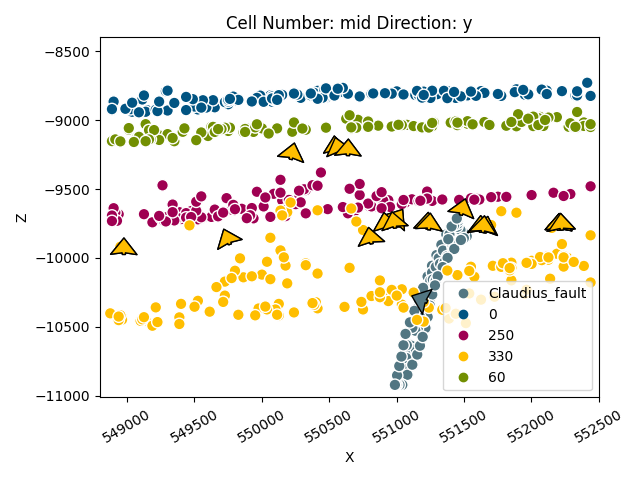Out:

<gempy.plot.visualization_2d.Plot2D object at 0x7fcc46042a60>


By default all surfaces belong to one unique series.

geo_model.surfaces

surface series order_surfaces color id
0 0 Default series 1 #015482 1
1 60 Default series 2 #9f0052 2
2 250 Default series 3 #ffbe00 3
3 330 Default series 4 #728f02 4
4 Claudius_fault Default series 5 #443988 5
5 basement Basement 1 #ff3f20 6

We will need to separate with surface belong to each series:

stratigraphy = 'fixed'

if stratigraphy == 'original':
gp.map_stack_to_surfaces(geo_model, {'Fault': 'Claudius_fault',
'Default series': ('0', '60', '250', '330'),
})
# Ordering the events from younger to older:
geo_model.reorder_series(['Fault', 'Default series', 'Basement'])

elif stratigraphy == 'fixed':
gp.map_stack_to_surfaces(geo_model, {'Default series': ('0', '60', '250'),
'Fault': 'Claudius_fault',
'Uncomformity': '330',
})
# Ordering the events from younger to older:
geo_model.reorder_series(['Default series', 'Fault', 'Uncomformity', 'Basement'])


So far we did not specify which series/faults are actula faults:

geo_model.set_is_fault('Fault')


Out:

Fault colors changed. If you do not like this behavior, set change_color to False.

order_series BottomRelation isActive isFault isFinite
Default series 1 Erosion True False False
Fault 2 Fault True True False
Uncomformity 3 Erosion True False False
Basement 4 Erosion False False False

Ordering the events from younger to older:

geo_model.reorder_series([‘Default series’, ‘Fault’, ‘Uncomformity’, ‘Basement’])

Check which series/faults are affected by other faults (rows offset columns):

geo_model.faults.faults_relations_df

Default series Fault Uncomformity Basement
Default series False False False False
Fault False False True True
Uncomformity False False False False
Basement False False False False

Now we are good to go:

gp.set_interpolator(geo_model, theano_optimizer='fast_run',
compile_theano=True)


Out:

Setting kriging parameters to their default values.
Compiling theano function...
Level of Optimization:  fast_run
Device:  cpu
Precision:  float64
Number of faults:  1
Compilation Done!
Kriging values:
values
range                 7047.13
$C_o$              1182430.95
drift equations  [3, 3, 3, 3]

<gempy.core.interpolator.InterpolatorModel object at 0x7fcc6b70cd60>

gp.compute_model(geo_model)


Out:

Lithology ids
[5. 5. 5. ... 1. 1. 1.]

sect = 

gp.plot_2d(geo_model, cell_number=sect, series_n=1, show_scalar=True, direction='x')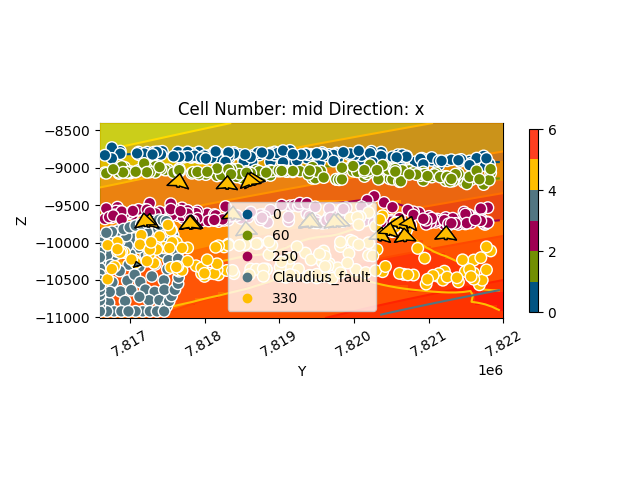Out:

<gempy.plot.visualization_2d.Plot2D object at 0x7fcc46bbdac0>

gp.plot_2d(geo_model, cell_number=sect, show_data=True, direction='x')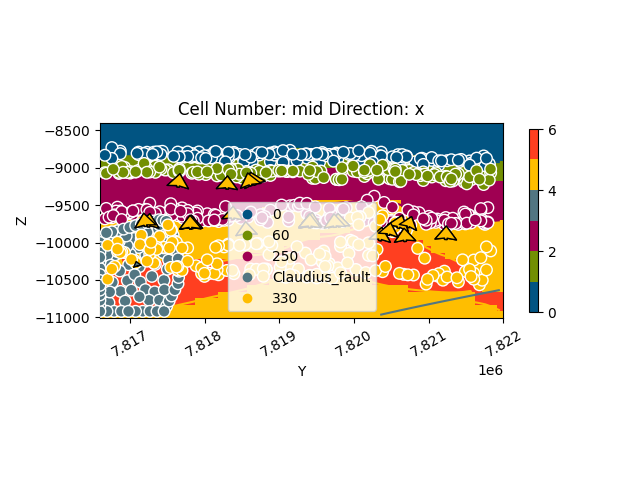Out:

<gempy.plot.visualization_2d.Plot2D object at 0x7fcc74890a60>

gp.plot_2d(geo_model, cell_number=, series_n=0, direction='y', show_scalar=True)
gp.plot_2d(geo_model, cell_number=, series_n=1, direction='y', show_scalar=True)
gp.plot_2d(geo_model, cell_number=, series_n=2, direction='y', show_scalar=True)

•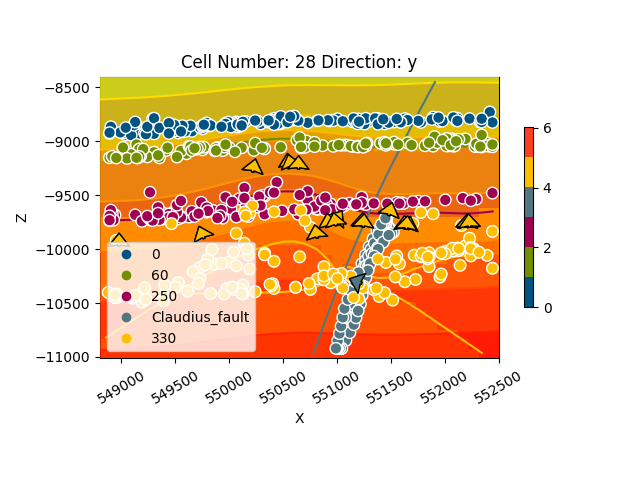•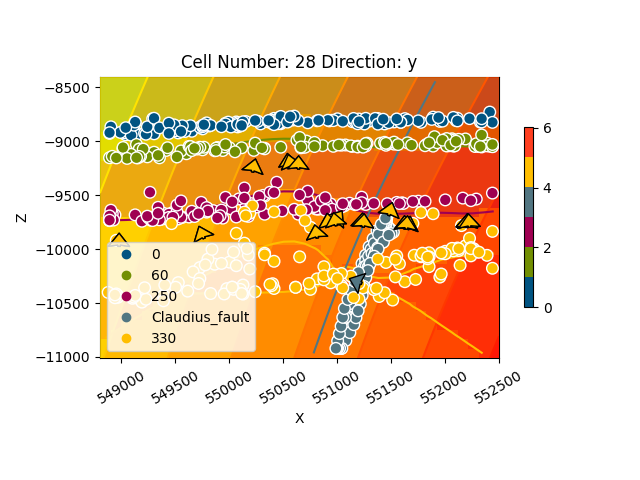•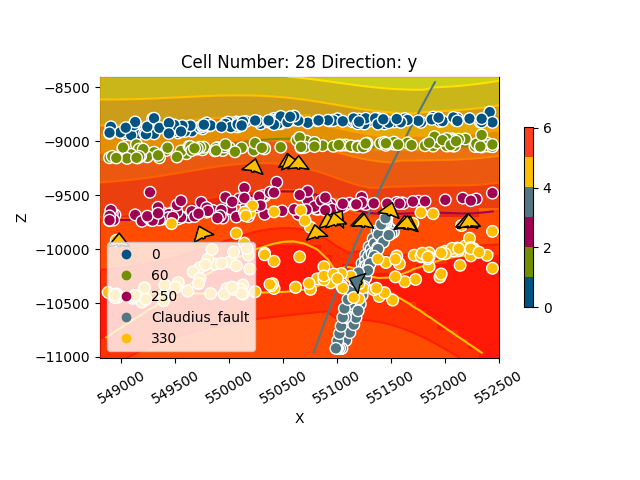Out:

<gempy.plot.visualization_2d.Plot2D object at 0x7fcc6b8d83a0>

gp.plot_2d(geo_model, cell_number=, show_data=True, direction='y')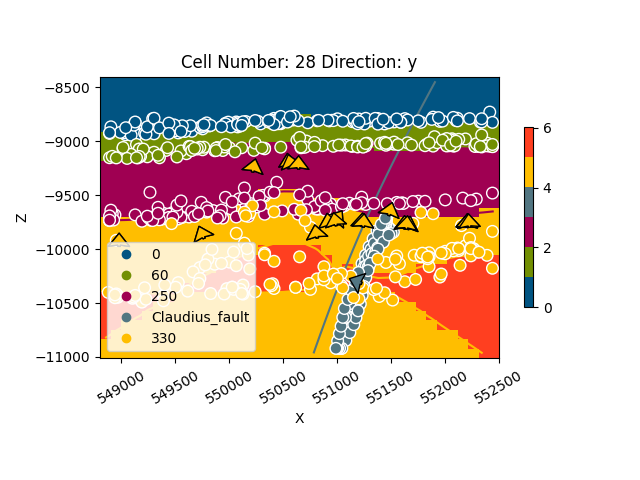Out:

<gempy.plot.visualization_2d.Plot2D object at 0x7fccd0e70df0>

# sphinx_gallery_thumbnail_number = 8
gp.plot_3d(geo_model)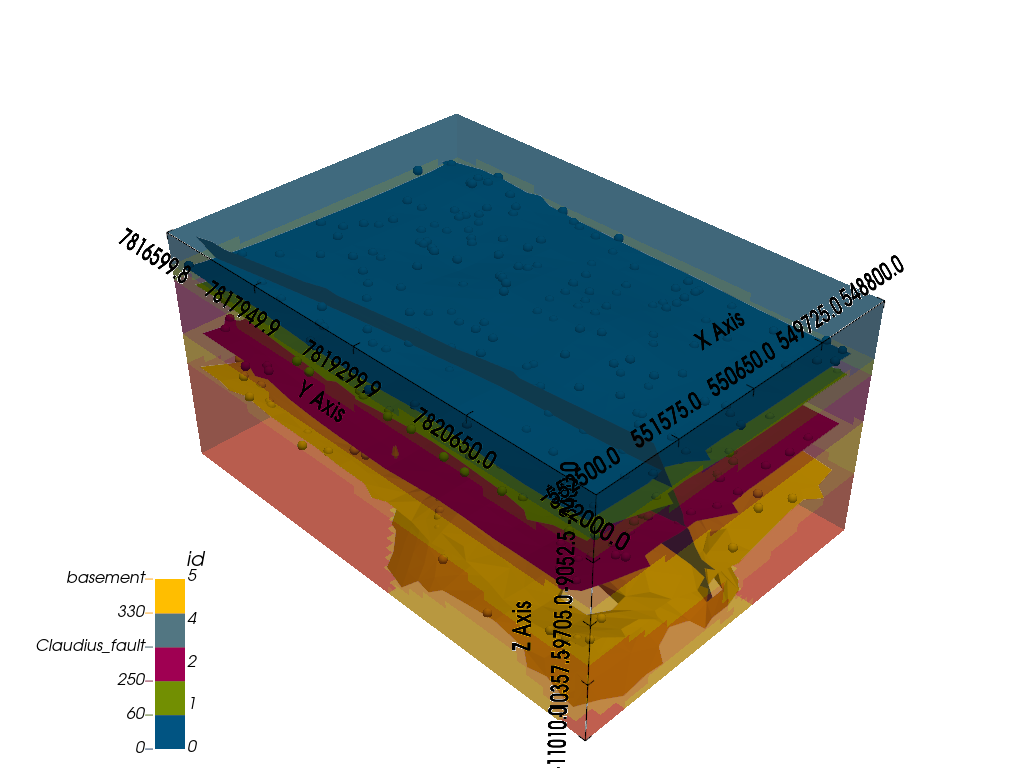Out:

<gempy.plot.vista.GemPyToVista object at 0x7fcb8ae53910>


# Export data:¶

The solution is stored in a numpy array of the following shape. Axis 0 are the scalar fields of each correspondent series/faults in the following order (except basement):

geo_model.series

order_series BottomRelation isActive isFault isFinite
Default series 1 Erosion True False False
Fault 2 Fault True True False
Uncomformity 3 Erosion True False False
Basement 4 Erosion False False False

For the surfaces, there are two numpy arrays, one with vertices and the other with triangles. Axis 0 is each surface in the order:

geo_model.surfaces

surface series order_surfaces color id
0 0 Default series 1 #015482 1
1 60 Default series 2 #9f0052 2
2 250 Default series 3 #ffbe00 3
4 Claudius_fault Fault 1 #527682 4
3 330 Uncomformity 1 #728f02 5
5 basement Basement 1 #ff3f20 6

np.save(‘Claudius_scalar’, geo_model.solutions.scalar_field_matrix) np.save(‘Claudius_ver’, geo_model.solutions.vertices) np.save(‘Claudius_edges’, geo_model.solutions.edges) gp.plot.export_to_vtk(geo_model, ‘Claudius’)

# Fault¶

• CPU Memory 8 Gb 44.9 s ± 150 ms per loop (mean ± std. dev. of 7 runs, 1 loop each)

• GPU Memory 6.8 gb:

• 2.13 s ± 3.39 ms per loop (mean ± std. dev. of 7 runs, 1 loop each) + steps str = [64.56394268] + steps str = [9927.69441126] + steps str = [196.15202667]

• 1.13 s ± 2.08 ms per loop (mean ± std. dev. of 7 runs, 1 loop each)

+ steps __str__ = [645.63943742]
+ steps __str__ = [99276.94573919]
+ steps __str__ = [1961.52029888]


def write_property_to_gocad_voxet(propertyfilename, propertyvalues):
"""
This function writes a numpy array into the right format for a gocad
voxet property file. This assumet there is a property already added to the .vo file,
and is just updating the file.
propertyfile - string giving the path to the file to write
propertyvalues - numpy array nz,ny,nx ordering and in float format
"""
propertyvalues = propertyvalues.astype('>f4')  # big endian
#     array = propertyvalues.newbyteorder()
propertyvalues.tofile(propertyfilename)

write_property_to_gocad_voxet('claudius_sf_gempy',
geo_model.solutions.scalar_field_matrix.reshape([38, 55, 30]).ravel('F'))

gp.save_model(geo_model)


Out:

True


Total running time of the script: ( 0 minutes 26.141 seconds)

Gallery generated by Sphinx-Gallery